### Category Theory

I've had a few people ask me questions about Adjunctions since my recent post and a request for some more introductory material, so I figured I would take a couple of short posts to tie Adjunctions to some other concepts.

Representable Functors

A covariant functor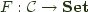is said to be representable by an object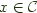if it is naturally isomorphic to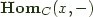.

We can translate that into Haskell, letting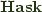play the role of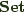with:

Grant B. asked me to post the derivation for the right and left Kan extension formula used in previous Kan Extension posts (1,2). For that we can turn to the definition of Kan extensions in terms of ends, but first we need to take a couple of steps back to find a way to represent (co)ends in Haskell.

I want to spend some more time talking about Kan extensions, composition of Kan extensions, and the relationship between a monad and the monad generated by a monad.

But first, I want to take a moment to recall adjunctions and show how they relate to some standard (co)monads, before tying them back to Kan extensions.

An adjunction between categories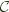and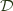consists of a pair of functors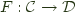, and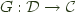and a natural isomorphism: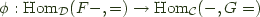We call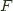the left adjoint functor, and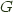the right adjoint functor and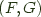an adjoint pair, and write this relationship as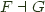I think I may spend a post or two talking about Kan extensions.

They appear to be black magic to Haskell programmers, but as Saunders Mac Lane said in Categories for the Working Mathematician:

All concepts are Kan extensions.

So what is a Kan extension? They come in two forms: right- and left- Kan extensions.

First I'll talk about right Kan extensions, since Haskell programmers have a better intuition for them.


> import Control.Arrow ((|||),(&&&),left)
> newtype Mu f = InF { outF :: f (Mu f) }


I want to talk about a novel recursion scheme that hasn't received a lot of attention from the Haskell community and its even more obscure dual -- which is necessarily more obscure because I believe this is the first time anyone has talked about it.

Jiri Adámek, Stefan Milius and Jiri Velebil have done a lot of work on Elgot algebras. Here I'd like to translate them into Haskell, dualize them, observe that the dual can encode primitive recursion, and provide some observations.

You can kind of think an Elgot algebra as a hylomorphism that cheats.


> elgot :: Functor f => (f b -> b) -> (a -> Either b (f a)) -> a -> b
> elgot phi psi = h where h = (id ||| phi . fmap h) . psi


The post below will only compile on a version of GHC >= 6.9, since it uses type families.

I did some digging and found the universal operations mentioned in the last couple of posts: unzip, unbizip and counzip were referenced as abide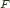, abideand coabide-- actually, I was looking for something else, and this fell into my lap.

They were apparently named for a notion defined by Richard Bird back in:

R.S. Bird. Lecture notes on constructive functional programming. In M. Broy, editor, Constructive Methods in Computing Science. International Summer School directed by F.L. Bauer [et al.], Springer Verlag, 1989. NATO Advanced Science Institute Series (Series F: Computer and System Sciences Vol. 55).

The notion can be summed up by defining that two binary operations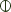and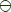abide if for all a, b, c, d: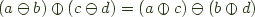.

There is a cute pictorial explanation of this idea in Maarten Fokkinga's remarkably readable Ph.D dissertation on p. 20.

The idea appears again on p.88 as part of the famous 'banana split' theorem, and then later on p90 the above names above are given along with the laws:


fmap f &&& fmap g = unfzip . fmap (f &&& g)
bimap f g &&& bimap h j = unbizip . bimap (f &&& h) (g &&& j)
fmap f ||| fmap g = fmap (f ||| g) . counfzip


That said the cases when the inverse operations exist do not appear to be mentioned in these sources.

Twan van Laarhoven pointed out that fzip from the other day is a close cousin of applicative chosen to be an inverse of the universal construction 'unfzip'.

During that post I also put off talking about the dual of zipping, so I figured I'd bring up the notion of choosing a notion of 'cozipping' by defining it as an inverse to a universally definable notion of 'counzipping'.


{-# OPTIONS -fglasgow-exts -fallow-undecidable-instances #-}
import Control.Arrow ((&&&), (***),(+++), (|||))


I want to talk about duality briefly. I don't want to go all the way to Filinski-style or Haskell is Not Not ML-style value/continuation duality, but I do want to poke a bit at the variant/record duality explified by the extensible cases used to handle variants in MLPolyR.

The need for extensible cases to handle open variants is part of the expression problem as stated by Wadler:

The goal is to define a data type by cases, where one can add new cases to the data type and new functions over the data type, without recompiling existing code, and while retaining static type safety.

One obvious trick is to use an extensible record of functions as a 'case' statement, with each field corresponding to one of the variants. To index into records you can use an extensible variant of functions to represent a field selection. In a purer form ala the Filinski or the Haskell is Not Not ML approach mentioned above, you can replace the word 'function' with continuation and everything works out.

Sweirstra recently tackled the extensible variant side of the equation with in Data types a la carte using the free monad coproduct to handle the 'variant' side of things, leaving the handling of cases to typeclasses, but we can see if we can go one better and just exploit the variant/record duality directly.

No, this isn't some uplifting piece about deriving courage from sloth in the face of adversity.

Transcribing the definition from category theory into Haskell we find that a strong monad is a functor such that there exists a morphism: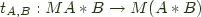with a couple of conditions on it that I'll get to later.

Currying that to get something that feels more natural to a Haskell programmer we get:


mstrength :: Monad m => m a -> b -> m (a,b)


Pardo provided us with a nice definition for that in Towards merging recursion and comonads:

In case it wasn't obvious, I thought I should mention that Kabanov and Vene's dynamorphisms which optimize histomorphisms for dynamic programming can be expressed readily as chronomorphisms; they just use an anamorphism instead of a futumorphism.

Back in the days of HYLO, it was common to write hylomorphisms with an additional natural transformation in them. Well, I was still coding in evil imperative languages back then, but I have it on reliable, er.. well supposition, that this is probably the case, or at least that they liked to do it back in the HYLO papers anyways.

Transcoding the category theory mumbo-jumbo into Haskell, so I can have a larger audience, we get the following 'frat combinator' -- you can blame Jules Bean from #haskell for that.


hyloEta :: Functor f =>
(g b -> b) ->
(forall a. f a -> g a) ->
(a -> f a)
hyloEta phi eta psi = phi . eta . fmap (hyloEta phi eta psi) . psi


We placed eta in the middle of the argument list because it is evocative of the fact that it occurs between phi and psi, and because that seems to be where everyone else puts it.

Now, clearly, we could roll eta into phi and get the more traditional hylo where f = g. Less obviously we could roll it into psi because it is a natural transformation and so the following diagram commutes:This 'Hylo Shift' property (mentioned in that same paper) allows us to move the 'eta' term into the phi term or into the psi term as we see fit. Since we can move the eta term around and it adds no value to the combinator, it quietly returned to the void from whence it came. hyloEta offers us no more power than hylo, so out it goes.

First, we can make the generalized hylomorphism from the other day more efficient by noting that once you inline the hylomorphism, you can see that you do 3 fmaps over the same structure, so we can fuse those together yielding:


(forall a. f (w a) -> w (f a)) ->
(forall a. m (f a) -> f (m a)) ->
(f (w b) -> b) ->
(a -> f (m a)) ->
(a -> b)
g_hylo w m f g = extract . g_hylo' w m f g . return

-- | the kernel of the generalized hylomorphism
(forall a. f (w a) -> w (f a)) ->
(forall a. m (f a) -> f (m a)) ->
(f (w b) -> b) ->
(a -> f (m a)) ->
(m a -> w b)
g_hylo' w m f g =
liftW f . w .
fmap (duplicate . g_hylo' w m f g . join) .
m . liftM g


Also, the above made me realize that most of the generalized cata/ana, etc morphisms give you a little more interesting stuff to do if you separate out the recursive part. Then you can pass it a monad built with something other than return to perform substitution on, or inspect the comonadic wrapper on the result.

Oh, and to support my earlier claim that g_hylo generalizes g_cata and g_ana here are derivations of each in terms of g_hylo.


g_cata :: (Functor f, Comonad w) =>
(forall a. f (w a) -> w (f a)) ->
(f (w a) -> a) ->
Mu f -> a

g_cata k f = g_hylo k (fmap Id . runId) f (fmap Id . outF)

g_ana :: (Functor f, Monad m) =>
(forall a. m (f a) -> f (m a)) ->
(a -> f (m a)) ->
a -> Nu f
g_ana k g = g_hylo (Id . fmap runId) k (InF . fmap runId) g


As an aside, histomorphisms have a dual that seems to be elided from most lists of recursion schemes: Uustalu and Vene call it a futumorphism. It basically lets you return a structure with seeds multiple levels deep rather than have to plumb 'one level at a time' through the anamorphism. While a histomorphism is a generalized catamorphism parameterized by the cofree comonad of your functor, a futumorphism is a generalized anamorphism parameterized by the free monad of your functor.


futu :: Functor f => (a -> f (Free f a)) -> a -> Nu f
futu f = ana ((f ||| id) . runFree) . return


Now, g_hylo is painfully general, so lets look at a particularly interesting choice of comonad and monad for a given functor that always have a distributive law: the cofree comonad, and the free monad of that very same functor!

This gives rise to a particular form of morphism that I haven't seem talked about in literature, which after kicking a few names around on the haskell channel we chose to call a chronomorphism because it subsumes histo- and futu- morphisms.


chrono :: Functor f =>
(f (Cofree f b) -> b) ->
(a -> f (Free f a)) ->
a -> b


Unlike most of the types of these generalized recursion schemes, chrono's type is quite readable!

A chronomorphism's fold operation can 'look back' at the results it has given, and its unfold operation can 'jump forward' by returning seeds nested multiple levels deep. It relies on the fact that you always have a distributive law for the cofree comonad of your functor over the functor itself and also one for the functor over its free monad and so it works for any Functor.

You can generalize it like you generalize histomorphisms and futumorphisms, and derive ana and catamorphisms from it by noting the fact that you can fmap extract or fmap return to deal with the cofree comonad or free monad parts of the term.

Alternately, since the 'identity comonad' can be viewed as the cofree comonad of the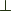Functor that maps everything to, you can also choose to rederive generalized futumorphisms from generalized chronomorphism using the distributive law of the identity comonad.

Below you'll find source code for generalized hylo- cata- ana- histo- futu- chrono- etc... morphisms and their separated kernels.

Source Code

As an aside, Dan Doel (dolio) has started packaging these up for addition to category-extras in Hackage.

I haven't seen written up anywhere the following operator (g_hylo), defined in the spirit of generalized catamorphisms and generalized anamorphisms, which seems to follow rather naturally from the definition of both -- I'm using liftW & liftM rather than fmap to make it clear what is being lifted over what.


class Functor w => Comonad w where
-- minimal definition: extend & extract or duplicate & extract
duplicate :: w a -> w (w a)
extend :: (w a -> b) -> w a -> w b
extract :: w a -> a
extend f = fmap f . duplicate
duplicate = extend id

liftW :: Comonad w => (a -> b) -> w a -> w b
liftW f = extend (f . extract)

(forall a. f (w a) -> w (f a)) ->
(forall a. m (f a) -> f (m a)) ->
(f (w b) -> b) ->
(a -> f (m a)) ->
a -> b
g_hylo w m f g =
extract .
hylo (liftW f . w . fmap duplicate) (fmap join . m . liftM g)
. return
where
hylo f g = f . fmap (hylo f g) . g


In the above, w and m are the distributive laws for the comonad and monad respectively, and hylo is a standard hylomorphism. In the style of Dave Menendez's Control.Recursion code it would be a 'refoldWith' and it can rederive a whole lot of recursion and corecursion patterns if not all of them.

Anyone?

You may recall the definition for an exponential functor from my previous entry, which can also be viewed, I suppose, as functors in the
category of right-invertible functions in Haskell.


class ExpFunctor f where
xmap :: (a -> b) -> (b -> a) -> f a -> f b


Clarifying the above, an instance of ExpFunctor should satisfy the slightly generalized version of the Functor laws from Control.Monad:


xmap id id = id
xmap f g . xmap f' g' = xmap (f . f') (g' . g)


Since we like to apply xmap to a pair of functions such that f . g = id, as in the Fegaras/Sheard case, we get:


xmap f g . xmap g f
= xmap (f . g) (f . g) -- by second xmap law
= xmap id id 	       -- by f . g = id
= id		       -- by first xmap law


In any event, what I thought what I'd do today is note that Wouter Swierstra's Data Types a la Carte approach works over exponential functors.

I have been trying out various representations for higher-order abstract syntax (HOAS) in Haskell, with an eye towards seeing what I can actually use to get real work done and I have run into a few unexpected headaches, and a couple of neat observations. That said, I should probably start by explaining some terminology.

Encoding a language that binds variables in higher order abstract syntax generally involves constructing an abstract data type that contains functions. A functor for representing expressions from Berendregdt's lambda cube in HOAS goes something like (ignoring any consolidation of binders and sorts)


data F a
= Lam a (a -> a)
| Pi a (a -> a)
| App a a
| Star
| Box


There are a number of mathematical functors that are not instances has Haskell's Functor class, such as the above.

« Previous Page